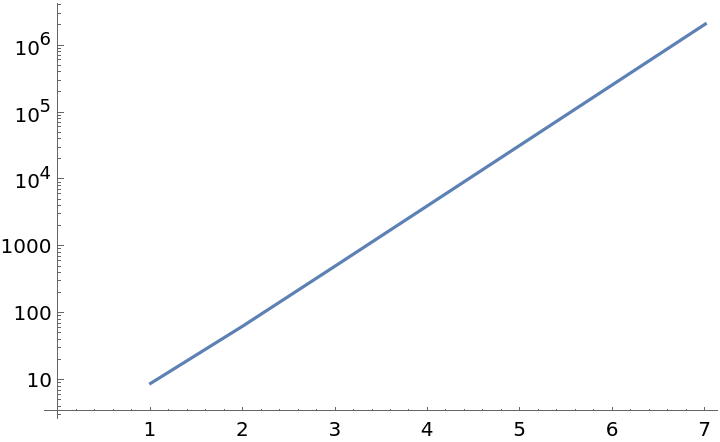Function Repository Resource:

MinkowskiSausageCurve

Generate the Minkowski sausage curve

Contributed by: Sander Huisman
 ResourceFunction["MinkowskiSausageCurve"][n] generates the line segments of the Minkowski sausage curve with n iterations. ResourceFunction["MinkowskiSausageCurve"][{p1,p2},n] uses the line connecting the points p1 and p2 as the initial line. ResourceFunction["MinkowskiSausageCurve"][{p1,p2,…,pn},n] uses the lines joining the points pi.

Details

The following transformation is done with each line segment: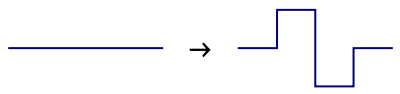The first 4 iterations: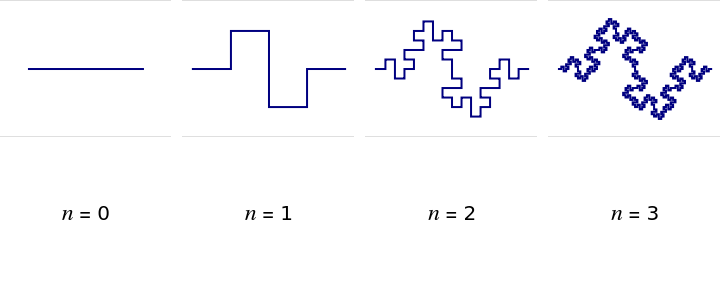ResourceFunction["MinkowskiSausageCurve"][n] is equivalent to ResourceFunction["MinkowskiSausageCurve"][{{-1,0},{1,0}},n]
The syntax ResourceFunction["MinkowskiSausageCurve"][Line[{p1,p2,,pn}],n] is also supported.

Examples

Basic Examples (2)

Generate the Minkowski sausage curve for 2 iterations:

 In:=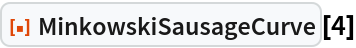Out=Generate the curve for 4 iterations and visualize the result:

 In:=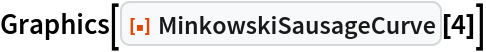Out=Scope (1)

Start from a square:

 In:=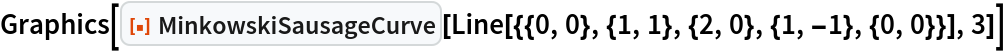Out=Properties and Relations (2)

MinkowskiSausageCurve and KochCurve have identical first iterates:

 In:=Out=Succeeding iterates have different behavior:

 In:=Out=The number of elements grows exponentially:

 In:=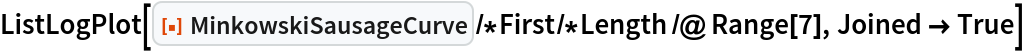Out=